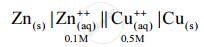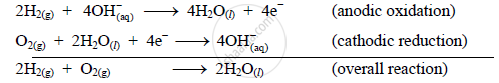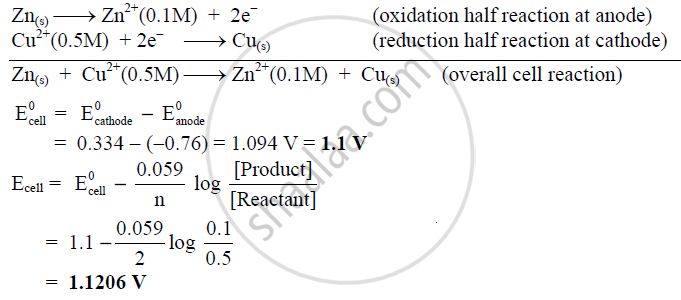HSC Science (Computer Science) 12th Board ExamMaharashtra State Board
Share

# Write Electrode Reaction and Net Cell Reaction for Fuel Cell. Calculate E.M.F. of the Following Cell at 25 °C - HSC Science (Computer Science) 12th Board Exam - Chemistry

#### Question

Write electrode reaction and net cell reaction for fuel cell. Calculate e.m.f. of the following cell at 25 °C.Standard reduction potential (SRP) of Zn and Cu are − 0.76 V and 0.334 V respectively

#### Solution

Cell reactions for fuel cell:

1) Oxidation at anode (−):

At anode, hydrogen gas is oxidized to H2O.

2H2(g) + 4OH_(aq)^- → 4H2O(l) + 4e

2) Reduction at cathode (+):

The electrons released at anode travel to cathode through the external circuit.
Here O2 gas is reduced to OH

O2(g) + 2H2O(l) + 4e → 4OH_((aq))^-

3) Net cell reaction

The overall cell reaction is the sum of oxidation at anode and reduction at cathode.
Thus,4) Calculation of e.m.f.:

E_(Cu)^0 = 0.334 V

E_(Zn)^0 = −0.76 V

The overall cell reaction is the redox reaction which is the sum of oxidation half reaction at anode and reduction half reaction at cathode.Is there an error in this question or solution?

#### APPEARS IN

2012-2013 (October) (with solutions)
Question 4.1 | 7.00 marks

#### Video TutorialsVIEW ALL 

Solution Write Electrode Reaction and Net Cell Reaction for Fuel Cell. Calculate E.M.F. of the Following Cell at 25 °C Concept: Batteries - fuel cell.
S